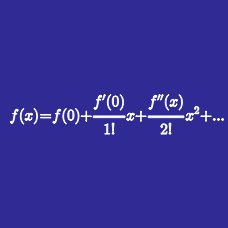Calculus

# Taylor Series: Level 5 Challenges

$f\left( x \right) =\sum _{ n=0 }^{ \infty }{ \frac { \sin { \left( xn \right) } }{ { 7 }^{ n } } }$ For all real numbers $x$, let $f(x)$ be a function with fundamental period $P$.

Let $\displaystyle a = \int_0^{P/2} f(x) \, dx$. If $f \left( \pi e^{|a|} \right)$ can be expressed as $-\dfrac{\alpha\sqrt \beta}{\gamma}$, where $\alpha, \beta$ and $\gamma$ are positive integers with $\alpha, \gamma$ coprime and $\beta$ square-free, find $\alpha + \beta + \gamma$.$\frac {1^7}{1!} + \frac {1^7 + 2^7}{2!} + \frac {1^7 + 2^7 + 3^7}{3!} + \frac {1^7 + 2^7 + 3^7 + 4^7}{4!} + \ldots$

If the series above equals to $W$, what is the value of $\frac {24}{e} \times W$?

Note: $e = \displaystyle \lim_{n \to \infty} \left (1 + \frac 1 n \right )^n$

Inspired by Caleb Townsend

###### Image Credit: Flickr Gingertwist

For all integers $n$, we define $\xi_n$ as follows: $\begin{cases} \xi_n = 1 & \text{if } n \equiv 0 \pmod{4} \text{ or } n \equiv 1 \pmod{4} \\ \xi_n= -1 & \text{if } n \equiv 2 \pmod{4} \text{ or } n \equiv 3 \pmod{4} \end{cases}$ For all $n \in \mathbb{Z^+}$, let $f(n)= \xi_0 \dbinom{n}{0} + \xi_1 \dbinom{n}{1} + \xi_2 \dbinom{n}{2} + \cdots + \xi_n \dbinom{n}{n}.$ Find $\left \lfloor 100 \left( \displaystyle \sum \limits_{n=0}^{\infty} \dfrac{f(n)}{n!} \right) \right \rfloor$.

Details and assumptions

• As an explicit example, since $4 \equiv 0 \pmod{4}$, $\xi_4= 1$, whereas $\xi_6 = -1$ since $6 \equiv 2 \pmod{4}$. Note that $\xi_0= \xi_1= 1$.

• The floor function $\lfloor x \rfloor$ denotes the largest integer less than or equal to $x$. For example, $\lfloor 3.25 \rfloor = 3, \lfloor 4 \rfloor= 4, \lfloor \pi \rfloor = 3$.

• You might use a scientific calculator for this problem.

$\large\displaystyle \lim_{x \to 0} \frac {(\cos x)^{\sin x} - \sqrt{1-x^3}}{x^6} =\ ?$

Find $\dfrac{f^{(2016)}(0)}{2016!}$ for $f(x)=\dfrac{x^3}{(1-x)^3(1+x+x^2)}$.

Clarifications:

• $f^{(k)}(x)$ denotes the $k^\text{th}$ derivative of $f(x)$.

• $!$ denotes the factorial notation. For example, $8! = 1\times2\times3\times\cdots\times8$.

×